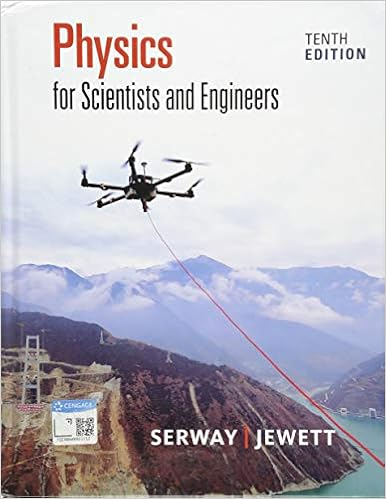# 8 earth has a density of 55 gcm 3 does earth contain

• Homework Help
• larissaanndeherz
• 5
• 97% (70) 68 out of 70 people found this document helpful

This preview shows page 1 - 5 out of 5 pages.

##### We have textbook solutions for you!
The document you are viewing contains questions related to this textbook.The document you are viewing contains questions related to this textbook.
Chapter 1 / Exercise 1
Physics for Scientists and Engineers
Jewett/SerwayExpert Verified
8. Earth has a density of 5.5 g/cm 3 . Does Earth contain something more dense than rock? (a) What could that substance be? (Hint: It is a relatively common but fairly dense element.) (b) Where is that substance mostly found on planet Earth? (Hint: The Earth is composed of several layers or regions. This substance is mostly found in one of those layers.) (a) _________________________________ [2 pts] (b) _________________________________ [2 pts] Larissa Anndeherz Europa and IO Mimas and Rhea Iron This substance is found in the deepest layer of earth. *See the detailed hints on page 5. Text
##### We have textbook solutions for you!
The document you are viewing contains questions related to this textbook.The document you are viewing contains questions related to this textbook.
Chapter 1 / Exercise 1
Physics for Scientists and Engineers
Jewett/SerwayExpert Verified
Activity #7 2 [Table = 39 pts] [Table = 18 pts] Planet Moon Density (g/cm 3 ) % Rock Jupiter Io 3.5 Europa 3.0 Ganymede 1.9 Callisto 1.8 Saturn Mimas 1.2 Dione 1.4 Rhea 1.3 Titan 1.9 Uranus Miranda 1.4 Ariel 1.7 Umbriel 1.5 Neptune Triton 2.1 Pluto Charon 2.0 Percent Rock Percent Ice Density of Moon 100 0 80 20 60 40 40 60 20 80 0 100 Note: A body cannot be composed of more than 100% of a substance. Note: Round the values for the densities calculated in Column 3 to 1 decimal. Note: The values for “% Rock” in this table are obtained directly from the graph plotted on page 3. Estimate these values to the nearest whole number. 3 2.5 1.7 1.3 .9 2.1
Activity #7 3 Rather than employ the graphical method to determine the percentage of rock in just one moon, your can use algebra. For example, what is the percentage of rock in Jupiter’s moon Callisto? From our table, see that Callisto has a density 1.8 g/cm 3 . We know the densities of rock and ice: D rock = 3.0 g/cm 3 ; D ice = 0.9 g/cm 3 . We can alter the equation found on page 1 to read: ࠵? Moon = 3.0࠵? + 0.9࠵? 100 D is the density of the moon, x is the percentage of rock, and y is the percentage of ice. (The 100 is there to convert the percentages to fractions.) The constants 3.0 and 0.9 are simply the densities of rock and ice, respectively. The problem is that we have one equation with two unknowns: x and y . This dilemma can be solved by a substitution. Because we have only two components, rock and ice, the percentage of one is 100 minus the percentage of the other. Thus, we have the following: ࠵? = 100 − ࠵? 0.9 [Graph = 20 pts] Note : For full credit, ALL points must be plotted. First, plot all six points. Then connect the first point and the last point with a line drawn with a ruler or suitable straight edge. This will be your best-fit line. Grade points will be deducted for a best-fit line that is not drawn using a ruler.
Activity #7 4 Substitute this into our main equation: ࠵? Moon = [3.0࠵? + 0.9(100 − ࠵?)] 100
•••